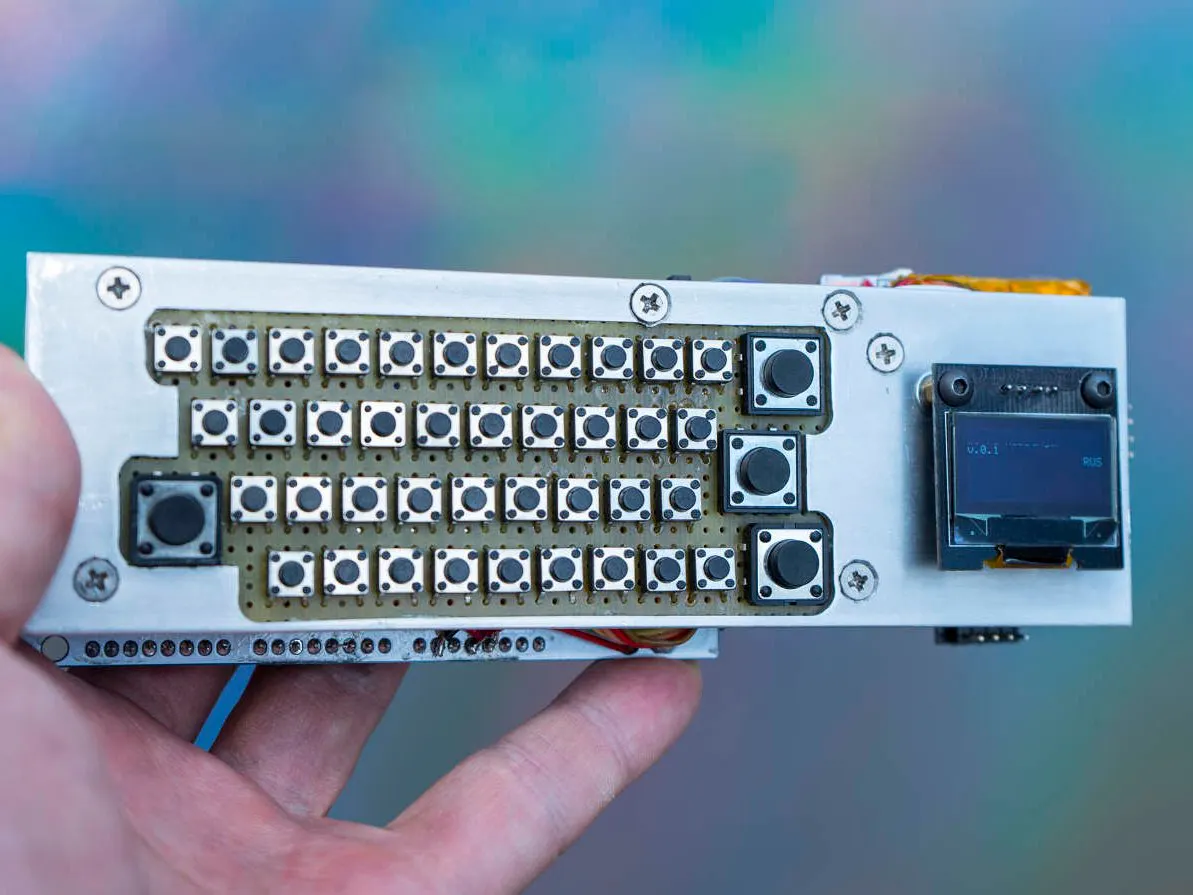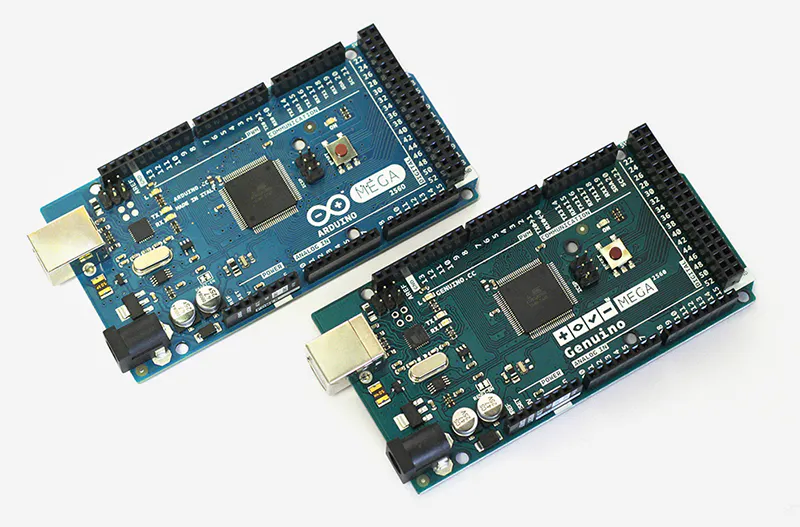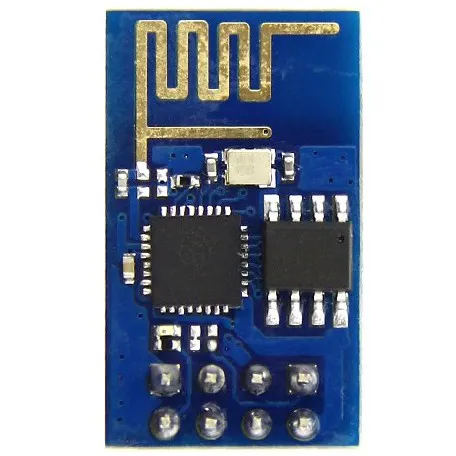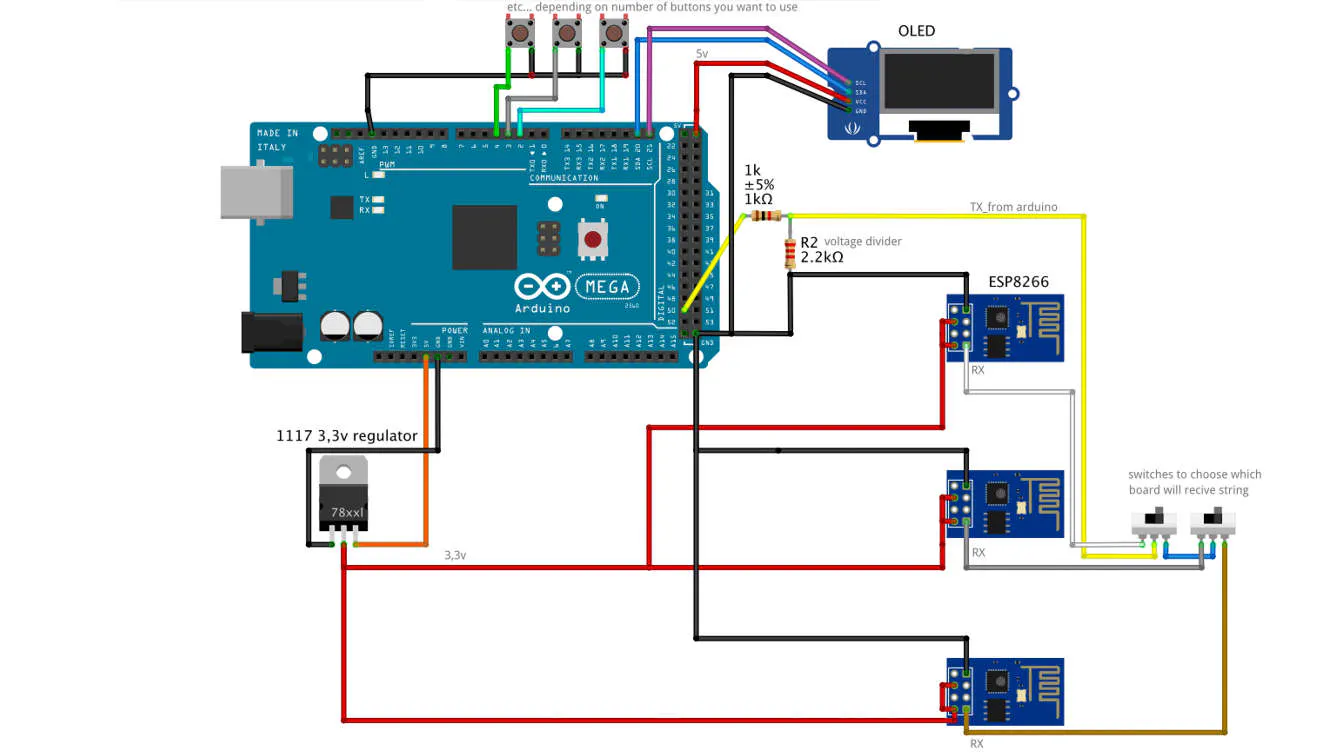Published

# ::vtol:: Hot Ninja

Multifunctional network device for autonomous activity in the city environment. Its main function is communication through Wi-Fi networks.

IntermediateProtip13,749## Things used in this project

### Hardware componentsArduino Mega 2560 & Genuino Mega 2560
×1Espressif ESP8266 ESP-01
×3

## Schematics

### basic idea## Code

### code for esp8266

Arduino
```#include <FS.h>
#include <ESP8266WiFi.h>
#include <DNSServer.h>
#include <ESP8266WebServer.h>
#include <PString.h>
#include <Streaming.h>

const IPAddress apIp(192, 168, 0, 1);
DNSServer dnsServer;
ESP8266WebServer server(80);

struct PrintMac
{
const uint8_t* mac;
};

Print&
operator<<(Print& p, const PrintMac& pmac)
{
const uint8_t* mac = pmac.mac;
for (int i = 0; i < 6; ++i) {
if (i > 0) {
p << ':';
}
int b = mac[i];
if (b < 0x10) {
p << '0';
}
p << _HEX(b);
}
return p;
}

File logfile; //create log files
File logfile1;
char logbuf;
PString logline(logbuf, sizeof(logbuf));
PString logline1(logbuf, sizeof(logbuf));
void
appendLog()
{
Serial << millis() << ' ' << logline << '\n';
logfile << millis() << ' ' << logline << '\n';
logline.begin();
}

void
appendLog1()
{
Serial << millis() << ' ' << logline1 << '\n';
logfile1 << millis() << ' ' << logline1 << '\n';
logline1.begin();
}

void
wifiStaConnect(const WiFiEventSoftAPModeStationConnected& evt) //start collecting log data
{
logline << PrintMac{evt.mac} << " assoc";
appendLog();
}
WiFiEventHandler wifiStaConnectHandler;

void
{
logline << PrintMac{evt.mac} << " deassoc";
appendLog();
}

void
httpDefault()
{
logline << server.client().remoteIP() << " redirect";
appendLog();
server.send(302, "text/plain", "");
server.client().stop();
}

void
httpHome()
{
return httpDefault();
}

logline << server.client().remoteIP() << " home";
appendLog();
File file = SPIFFS.open("/index.htm.gz", "r");
server.streamFile(file, "text/html");
file.close();
}

void
httpConnect()
{
logline << server.client().remoteIP() << " /// message: " << server.arg("email"); //if message is send then it's redirected to log2.txt
appendLog1();
File file = SPIFFS.open("/connect.htm.gz", "r");
server.streamFile(file, "text/html");
file.close();
}

void
httpPureCss()
{
File file = SPIFFS.open("/pure.css.gz", "r");
server.streamFile(file, "text/css");
file.close();
}

void
httpLog()
{
logline << server.client().remoteIP() << " log";
appendLog();
logfile.seek(0, SeekSet);
server.streamFile(logfile, "text/plain; charset=UTF-8");
logfile.seek(0, SeekEnd);
}

void
httpLog1() //message goes to log2.txt file
{

appendLog1();
logfile1.seek(0, SeekSet);
server.streamFile(logfile1, "text/plain; charset=UTF-8"); //set UTF-8 for both latin and cyrilic charters of messages
logfile1.seek(0, SeekEnd);
}

void
setup()
{
Serial.begin(115200);
Serial.println();

SPIFFS.begin();
logfile = SPIFFS.open("/log.txt", "a+");
logfile1 = SPIFFS.open("/log1.txt", "a+"); //SPIFFS memory files

WiFi.persistent(false);
WiFi.disconnect(true);
wifiStaConnectHandler = WiFi.onSoftAPModeStationConnected(wifiStaConnect);
WiFi.mode(WIFI_AP);
WiFi.softAPConfig(apIp, apIp, IPAddress(255, 255, 255, 0));
WiFi.softAP("MT_FREE", nullptr, 1); //const network name wich will be changed after recieving data from serial input

dnsServer.start(53, "*", apIp);

server.on("/", httpHome);
server.on("/connect", httpConnect);
server.on("/pure.css", httpPureCss);
server.on("/log.txt", httpLog); //main log file
server.on("/log1.txt", httpLog1); //messages file
server.onNotFound(httpDefault);
server.begin();

}

void
loop()
{
dnsServer.processNextRequest();
server.handleClient();

if (Serial.available() > 0) {
String text = Serial.readString(); //read incomming string to rename the softAP (network name)
char copy;
text.toCharArray(copy, 50);
WiFiUDP Udp;
char packetBuffer;
unsigned int localPort = 9999;
Serial.begin(115200);
WiFi.softAP(copy);
Udp.begin(localPort);

}
}
```

### code for arduino mega

Arduino
```#include <iarduino_OLED_txt.h>     //oled lib. could be any related to your OLED
iarduino_OLED_txt myOLED(0x78);    //oled lib. could be any related to your OLED
extern uint8_t SmallFontRus[];     // if you need cyrilic
String stringOne, stringTwo, stringThree, stringFour; // strings for messages, don't remember if I use all of them or just 2
char packetBuffer;
#include <SoftwareSerial.h> //soft serial to connect MEGA with esp2866
SoftwareSerial mySerial(50, 51); //soft serial to pins
unsigned int lastStringLength = stringThree.length(); //counting string length to cut it of needed

const int buttonPin2 = 2; // keyboard buttons pins:
const int buttonPin3 = 3;
const int buttonPin4 = 4;
const int buttonPin5 = 5;
const int buttonPin6 = 6;
const int buttonPin7 = 7;
const int buttonPin8 = 8;
const int buttonPin9 = 9;
const int buttonPin10 = 10;
const int buttonPin11 = 11;
const int buttonPin12 = 12;
const int buttonPin13 = 13;
const int buttonPin14 = 14;
const int buttonPin15 = 15;
const int buttonPin16 = 16;
const int buttonPin17 = 17;
const int buttonPin18 = 18;
const int buttonPin19 = 19;
const int buttonPin20 = 45;
const int buttonPin21 = 46;
const int buttonPin22 = 22;
const int buttonPin23 = 23;
const int buttonPin24 = 24;
const int buttonPin25 = 25;
const int buttonPin26 = 26;
const int buttonPin27 = 27;
const int buttonPin28 = 28;
const int buttonPin29 = 29;
const int buttonPin30 = 30;
const int buttonPin31 = 31;
const int buttonPin32 = 32;
const int buttonPin33 = 33;
const int buttonPin34 = 34;
const int buttonPin35 = 35;
const int buttonPin36 = 36;
const int buttonPin37 = 37;
const int buttonPin38 = 38;
const int buttonPin39 = 39;
const int buttonPin40 = 40;
const int buttonPin41 = 41;
const int buttonPin42 = 42;
const int buttonPin43 = 43;
const int buttonPin44 = 44;
const int buttonPin52 = 52; //not liniar as you seen, I don't remember why, maybe because it was more easy to solder it like this.
const int buttonPin53 = 53;

int val; // variable used for debugging and detecting buttons presses in serial monitor (not softserial), not really needed but could be usefull if you have your own setup of buttons

int buttonState2 = 0;
int buttonState3 = 0;
int buttonState4 = 0;
int buttonState5 = 0;
int buttonState6 = 0;
int buttonState7 = 0;
int buttonState8 = 0;
int buttonState9 = 0;
int buttonState10 = 0;
int buttonState11 = 0;
int buttonState12 = 0;
int buttonState13 = 0;
int buttonState14 = 0;
int buttonState15 = 0;
int buttonState16 = 0;
int buttonState17 = 0;
int buttonState18 = 0;
int buttonState19 = 0;
int buttonState20 = 0;
int buttonState21 = 0;
int buttonState22 = 0;
int buttonState23 = 0;
int buttonState24 = 0;
int buttonState25 = 0;
int buttonState26 = 0;
int buttonState27 = 0;
int buttonState28 = 0;
int buttonState29 = 0;
int buttonState30 = 0;
int buttonState31 = 0;
int buttonState32 = 0;
int buttonState33 = 0;
int buttonState34 = 0;
int buttonState35 = 0;
int buttonState36 = 0;
int buttonState37 = 0;
int buttonState38 = 0;
int buttonState39 = 0;
int buttonState40 = 0;
int buttonState41 = 0;
int buttonState42 = 0;
int buttonState43 = 0;
int buttonState44 = 0;
int buttonState52 = 0;
int buttonState53 = 0;

void setup() {

myOLED.begin();
myOLED.setFont(SmallFontRus);

Serial.begin(115200);
mySerial.begin(115200);

pinMode(buttonPin2, INPUT);
pinMode(buttonPin3, INPUT);
pinMode(buttonPin4, INPUT);
pinMode(buttonPin5, INPUT);
pinMode(buttonPin6, INPUT);
pinMode(buttonPin7, INPUT);
pinMode(buttonPin8, INPUT);
pinMode(buttonPin9, INPUT);
pinMode(buttonPin10, INPUT);
pinMode(buttonPin11, INPUT);
pinMode(buttonPin12, INPUT);
pinMode(buttonPin13, INPUT);
pinMode(buttonPin14, INPUT);
pinMode(buttonPin15, INPUT);
pinMode(buttonPin16, INPUT);
pinMode(buttonPin17, INPUT);
pinMode(buttonPin18, INPUT);
pinMode(buttonPin19, INPUT);
pinMode(buttonPin20, INPUT);
pinMode(buttonPin21, INPUT);
pinMode(buttonPin22, INPUT);
pinMode(buttonPin23, INPUT);
pinMode(buttonPin24, INPUT);
pinMode(buttonPin25, INPUT);
pinMode(buttonPin26, INPUT);
pinMode(buttonPin27, INPUT);
pinMode(buttonPin28, INPUT);
pinMode(buttonPin29, INPUT);
pinMode(buttonPin30, INPUT);
pinMode(buttonPin31, INPUT);
pinMode(buttonPin32, INPUT);
pinMode(buttonPin33, INPUT);
pinMode(buttonPin34, INPUT);
pinMode(buttonPin35, INPUT);
pinMode(buttonPin36, INPUT);
pinMode(buttonPin37, INPUT);
pinMode(buttonPin38, INPUT);
pinMode(buttonPin39, INPUT);
pinMode(buttonPin40, INPUT);
pinMode(buttonPin41, INPUT);
pinMode(buttonPin42, INPUT);
pinMode(buttonPin43, INPUT);
pinMode(buttonPin44, INPUT);
pinMode(buttonPin52, INPUT);
pinMode(buttonPin53, INPUT);

digitalWrite(2, HIGH); //pull-up pins, so you don't need any resistors (much less soldering).
digitalWrite(3, HIGH);
digitalWrite(4, HIGH);
digitalWrite(5, HIGH);
digitalWrite(6, HIGH);
digitalWrite(7, HIGH);
digitalWrite(8, HIGH);
digitalWrite(9, HIGH);
digitalWrite(10, HIGH);
digitalWrite(11, HIGH);
digitalWrite(12, HIGH);
digitalWrite(13, HIGH);
digitalWrite(14, HIGH);
digitalWrite(15, HIGH);
digitalWrite(16, HIGH);
digitalWrite(17, HIGH);
digitalWrite(18, HIGH);
digitalWrite(19, HIGH);
digitalWrite(45, HIGH);
digitalWrite(46, HIGH);
digitalWrite(22, HIGH);
digitalWrite(23, HIGH);
digitalWrite(24, HIGH);
digitalWrite(25, HIGH);
digitalWrite(26, HIGH);
digitalWrite(27, HIGH);
digitalWrite(28, HIGH);
digitalWrite(29, HIGH);
digitalWrite(30, HIGH);
digitalWrite(31, HIGH);
digitalWrite(32, HIGH);
digitalWrite(33, HIGH);
digitalWrite(34, HIGH);
digitalWrite(35, HIGH);
digitalWrite(36, HIGH);
digitalWrite(37, HIGH);
digitalWrite(38, HIGH);
digitalWrite(39, HIGH);
digitalWrite(40, HIGH);
digitalWrite(41, HIGH);
digitalWrite(42, HIGH);
digitalWrite(43, HIGH);
digitalWrite(44, HIGH);
digitalWrite(52, HIGH);
digitalWrite(53, HIGH);
}

void loop() {

/////////////////// eng charters

if (buttonState53 == LOW) {     //switcher that is choosing the language is in "ENG" position, from now all the charters will be latin.
myOLED.print("ENG", OLED_R, 3);

if (buttonState2 == LOW) {
stringOne = " "; //add "space" to string
stringTwo = stringThree + stringOne;
delay (1);
stringThree = stringTwo;
val = 2;
Serial.println(val);
delay (151); //delay to make it singleshot
}

if (buttonState3 == LOW) {     //ENTER that sends string to the esp2866
char copy;
stringThree.toCharArray(copy, 32);
val = 3;
Serial.println(val);
mySerial.println(copy);
myOLED.print("send", OLED_R, 7);
delay (1000);
myOLED.print("        ", OLED_R, 7);
}

if (buttonState4 == LOW) {     //delet last charter from the string
stringThree = stringThree.substring(0, stringThree.length() - 1);
val = 4;
Serial.println(val);
delay (50);
myOLED.print("                                                ", 0, 5); //clean lines on OLED
myOLED.print("                                                ", 0, 6); //clean lines on OLED
}

if (buttonState5 == HIGH) {     //shift key is not pressed, all charters are small
myOLED.print("        ", 0, 7); //clean the line where the "shift" should be writen

if (buttonState6 == LOW) {
stringOne = "1";
stringTwo = stringThree + stringOne;
delay (1);
stringThree = stringTwo;
val = 6;
Serial.println(val);
delay (151);
}

if (buttonState7 == LOW) {

stringOne = "2";
stringTwo = stringThree + stringOne;
delay (1);
stringThree = stringTwo;
val = 7;
Serial.println(val);
delay (151);

}

if (buttonState8 == LOW) {
stringOne = "3";
stringTwo = stringThree + stringOne;
delay (1);
stringThree = stringTwo;
val = 8;
Serial.println(val);
delay (151);

}

if (buttonState9 == LOW) {
stringOne = "4";
stringTwo = stringThree + stringOne;
delay (1);
stringThree = stringTwo;
val = 9;
Serial.println(val);
delay (151);

}

if (buttonState10 == LOW) {
stringOne = "5";
stringTwo = stringThree + stringOne;
delay (1);
stringThree = stringTwo;
val = 10;
Serial.println(val);
delay (151);
}

if (buttonState11 == LOW) {
stringOne = "6";
stringTwo = stringThree + stringOne;
delay (1);
stringThree = stringTwo;
val = 12;
val = 11;
Serial.println(val);
delay (151);

}

if (buttonState12 == LOW) {
stringOne = "7";
stringTwo = stringThree + stringOne;
delay (1);
stringThree = stringTwo;
val = 12;
Serial.println(val);
delay (151);

}

if (buttonState13 == LOW) {
stringOne = "8";
stringTwo = stringThree + stringOne;
delay (1);
stringThree = stringTwo;
val = 13;
Serial.println(val);
delay (151);

}

if (buttonState14 == LOW) {
stringOne = "9";
stringTwo = stringThree + stringOne;
delay (1);
stringThree = stringTwo;
val = 14;
Serial.println(val);
delay (151);
}

if (buttonState15 == LOW) {
stringOne = "0";
stringTwo = stringThree + stringOne;
delay (1);
stringThree = stringTwo;
val = 15;
Serial.println(val);
delay (151);

}

if (buttonState16 == LOW) {
stringOne = "-";
stringTwo = stringThree + stringOne;
delay (1);
stringThree = stringTwo;
val = 16;
Serial.println(val);
delay (151);

}

if (buttonState17 == LOW) {
stringOne = "q";
stringTwo = stringThree + stringOne;
delay (1);
stringThree = stringTwo;
val = 17;
Serial.println(val);
delay (151);

}

if (buttonState18 == LOW) {
stringOne = "w";
stringTwo = stringThree + stringOne;
delay (1);
stringThree = stringTwo;
val = 18;
Serial.println(val);
delay (151);
}

if (buttonState19 == LOW) {
stringOne = "e";
stringTwo = stringThree + stringOne;
delay (1);
stringThree = stringTwo;
val = 19;
Serial.println(val);
delay (151);

}

if (buttonState20 == LOW) {
stringOne = "r";
stringTwo = stringThree + stringOne;
delay (1);
stringThree = stringTwo;
val = 20;
Serial.println(val);
delay (151);

}

if (buttonState21 == LOW) {
stringOne = "t";
stringTwo = stringThree + stringOne;
delay (1);
stringThree = stringTwo;
val = 21;
Serial.println(val);
delay (151);

}

if (buttonState22 == LOW) {
stringOne = "y";
stringTwo = stringThree + stringOne;
delay (1);
stringThree = stringTwo;
val = 22;
Serial.println(val);
delay (151);
}

if (buttonState23 == LOW) {
stringOne = "u";
stringTwo = stringThree + stringOne;
delay (1);
stringThree = stringTwo;
val = 23;
Serial.println(val);
delay (151);

}

if (buttonState24 == LOW) {
stringOne = "i";
stringTwo = stringThree + stringOne;
delay (1);
stringThree = stringTwo;
val = 24;
Serial.println(val);
delay (151);

}

if (buttonState25 == LOW) {
stringOne = "o";
stringTwo = stringThree + stringOne;
delay (1);
stringThree = stringTwo;
val = 25;
Serial.println(val);
delay (151);
}

if (buttonState26 == LOW) {
stringOne = "p";
stringTwo = stringThree + stringOne;
delay (1);
stringThree = stringTwo;
val = 26;
Serial.println(val);
delay (151);

}

if (buttonState27 == LOW) {
stringOne = "a";
stringTwo = stringThree + stringOne;
delay (1);
stringThree = stringTwo;
val = 27;
Serial.println(val);
delay (151);
}

if (buttonState28 == LOW) {
stringOne = "s";
stringTwo = stringThree + stringOne;
delay (1);
stringThree = stringTwo;
val = 28;
Serial.println(val);
delay (151);

}

if (buttonState29 == LOW) {
stringOne = "d";
stringTwo = stringThree + stringOne;
delay (1);
stringThree = stringTwo;
val = 29;
Serial.println(val);
delay (151);

}

if (buttonState30 == LOW) {
stringOne = "f";
stringTwo = stringThree + stringOne;
delay (1);
stringThree = stringTwo;
val = 30;
Serial.println(val);
delay (151);

}

if (buttonState31 == LOW) {
stringOne = "g";
stringTwo = stringThree + stringOne;
delay (1);
stringThree = stringTwo;
val = 31;
Serial.println(val);
delay (151);
}

if (buttonState32 == LOW) {
stringOne = "h";
stringTwo = stringThree + stringOne;
delay (1);
stringThree = stringTwo;
val = 32;
Serial.println(val);
delay (151);

}

if (buttonState33 == LOW) {
stringOne = "j";
stringTwo = stringThree + stringOne;
delay (1);
stringThree = stringTwo;
val = 33;
Serial.println(val);
delay (151);

}

if (buttonState34 == LOW) {
stringOne = "k";
stringTwo = stringThree + stringOne;
delay (1);
stringThree = stringTwo;
val = 34;
Serial.println(val);
delay (151);
}

if (buttonState35 == LOW) {
stringOne = "l";
stringTwo = stringThree + stringOne;
delay (1);
stringThree = stringTwo;
val = 35;
Serial.println(val);
delay (151);

}

if (buttonState36 == LOW) {
stringOne = "z";
stringTwo = stringThree + stringOne;
delay (1);
stringThree = stringTwo;
val = 36;
Serial.println(val);
delay (151);
}

if (buttonState37 == LOW) {
stringOne = "x";
stringTwo = stringThree + stringOne;
delay (1);
stringThree = stringTwo;
val = 37;
Serial.println(val);
delay (151);

}

if (buttonState38 == LOW) {
stringOne = "c";
stringTwo = stringThree + stringOne;
delay (1);
stringThree = stringTwo;
val = 38;
Serial.println(val);
delay (151);

}

if (buttonState39 == LOW) {
stringOne = "v";
stringTwo = stringThree + stringOne;
delay (1);
stringThree = stringTwo;
val = 39;
Serial.println(val);
delay (151);

}

if (buttonState40 == LOW) {
stringOne = "b";
stringTwo = stringThree + stringOne;
delay (1);
stringThree = stringTwo;
val = 40;
Serial.println(val);
delay (151);
}

if (buttonState41 == LOW) {
stringOne = "n";
stringTwo = stringThree + stringOne;
delay (1);
stringThree = stringTwo;
val = 41;
Serial.println(val);
delay (151);

}

if (buttonState42 == LOW) {
stringOne = "m";
stringTwo = stringThree + stringOne;
delay (1);
stringThree = stringTwo;
val = 42;
Serial.println(val);
delay (151);

}

if (buttonState43 == LOW) {
stringOne = ",";
stringTwo = stringThree + stringOne;
delay (1);
stringThree = stringTwo;
val = 43;
Serial.println(val);
delay (151);
}

if (buttonState44 == LOW) {
stringOne = ".";
stringTwo = stringThree + stringOne;
delay (1);
stringThree = stringTwo;
val = 44;
Serial.println(val);
delay (151);

}
}

// "no shift" end

if (buttonState5 == LOW) {     // with shift pressed:

myOLED.print("shift", 0, 7);
val = 5;
Serial.println(val);
delay (1);

if (buttonState6 == LOW) {
stringOne = "!";
stringTwo = stringThree + stringOne;
delay (1);
stringThree = stringTwo;
val = 6;
Serial.println(val);
delay (151);
}

if (buttonState7 == LOW) {
stringOne = "@";
stringTwo = stringThree + stringOne;
delay (1);
stringThree = stringTwo;
val = 7;
Serial.println(val);
delay (151);

}

if (buttonState8 == LOW) {

stringOne = "#";
stringTwo = stringThree + stringOne;
delay (1);
stringThree = stringTwo;
val = 8;
Serial.println(val);
delay (151);

}

if (buttonState9 == LOW) {
stringOne = "\$";
stringTwo = stringThree + stringOne;
delay (1);
stringThree = stringTwo;
val = 9;
Serial.println(val);
delay (151);

}

if (buttonState10 == LOW) {
stringOne = "%";
stringTwo = stringThree + stringOne;
delay (1);
stringThree = stringTwo;
val = 10;
Serial.println(val);
delay (151);
}

if (buttonState11 == LOW) {
stringOne = "^";
stringTwo = stringThree + stringOne;
delay (1);
stringThree = stringTwo;
val = 12;
val = 11;
Serial.println(val);
delay (151);

}

if (buttonState12 == LOW) {
stringOne = "&";
stringTwo = stringThree + stringOne;
delay (1);
stringThree = stringTwo;
val = 12;
Serial.println(val);
delay (151);

}

if (buttonState13 == LOW) {
stringOne = "*";
stringTwo = stringThree + stringOne;
delay (1);
stringThree = stringTwo;
val = 13;
Serial.println(val);
delay (151);

}

if (buttonState14 == LOW) {
stringOne = "(";
stringTwo = stringThree + stringOne;
delay (1);
stringThree = stringTwo;
val = 14;
Serial.println(val);
delay (151);
}

if (buttonState15 == LOW) {
stringOne = ")";
stringTwo = stringThree + stringOne;
delay (1);
stringThree = stringTwo;
val = 15;
Serial.println(val);
delay (151);

}

if (buttonState16 == LOW) {
stringOne = "_";
stringTwo = stringThree + stringOne;
delay (1);
stringThree = stringTwo;
val = 16;
Serial.println(val);
delay (151);

}

if (buttonState17 == LOW) {
stringOne = "Q";
stringTwo = stringThree + stringOne;
delay (1);
stringThree = stringTwo;
val = 17;
Serial.println(val);
delay (151);

}

if (buttonState18 == LOW) {
stringOne = "W";
stringTwo = stringThree + stringOne;
delay (1);
stringThree = stringTwo;
val = 18;
Serial.println(val);
delay (151);
}

if (buttonState19 == LOW) {
stringOne = "E";
stringTwo = stringThree + stringOne;
delay (1);
stringThree = stringTwo;
val = 19;
Serial.println(val);
delay (151);

}

if (buttonState20 == LOW) {
stringOne = "R";
stringTwo = stringThree + stringOne;
delay (1);
stringThree = stringTwo;
val = 20;
Serial.println(val);
delay (151);

}

if (buttonState21 == LOW) {
stringOne = "T";
stringTwo = stringThree + stringOne;
delay (1);
stringThree = stringTwo;
val = 21;
Serial.println(val);
delay (151);

}

if (buttonState22 == LOW) {
stringOne = "Y";
stringTwo = stringThree + stringOne;
delay (1);
stringThree = stringTwo;
val = 22;
Serial.println(val);
delay (151);
}

if (buttonState23 == LOW) {
stringOne = "U";
stringTwo = stringThree + stringOne;
delay (1);
stringThree = stringTwo;
val = 23;
Serial.println(val);
delay (151);

}

if (buttonState24 == LOW) {
stringOne = "I";
stringTwo = stringThree + stringOne;
delay (1);
stringThree = stringTwo;
val = 24;
Serial.println(val);
delay (151);

}

if (buttonState25 == LOW) {
stringOne = "O";
stringTwo = stringThree + stringOne;
delay (1);
stringThree = stringTwo;
val = 25;
Serial.println(val);
delay (151);
}

if (buttonState26 == LOW) {
stringOne = "P";
stringTwo = stringThree + stringOne;
delay (1);
stringThree = stringTwo;
val = 26;
Serial.println(val);
delay (151);

}

if (buttonState27 == LOW) {
stringOne = "A";
stringTwo = stringThree + stringOne;
delay (1);
stringThree = stringTwo;
val = 27;
Serial.println(val);
delay (151);
}

if (buttonState28 == LOW) {
stringOne = "S";
stringTwo = stringThree + stringOne;
delay (1);
stringThree = stringTwo;
val = 28;
Serial.println(val);
delay (151);

}

if (buttonState29 == LOW) {
stringOne = "D";
stringTwo = stringThree + stringOne;
delay (1);
stringThree = stringTwo;
val = 29;
Serial.println(val);
delay (151);

}

if (buttonState30 == LOW) {
stringOne = "F";
stringTwo = stringThree + stringOne;
delay (1);
stringThree = stringTwo;
...